# Mathematics Mock Test - 1

## 20 Questions MCQ Test Mathematics for Class 5: NCERT | Mathematics Mock Test - 1

Description
Attempt Mathematics Mock Test - 1 | 20 questions in 20 minutes | Mock test for Class 5 preparation | Free important questions MCQ to study Mathematics for Class 5: NCERT for Class 5 Exam | Download free PDF with solutions
QUESTION: 1

### Which part of the circle does segment U represent?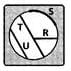Solution:

A chord of a circle divides the circle into two regions, which are called the segments of the circle. The minor segment is the region bounded by the chord and the minor arc intercepted by the chord. The major segment is the region bounded by the chord and the major arc intercepted by the chord.

QUESTION: 2

Solution:

1/5+2/5 = 3/5

QUESTION: 3

### Find the area of the shaded part.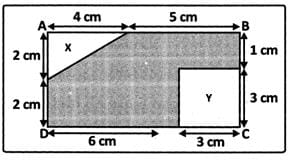Solution: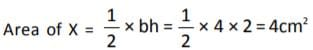Area of Y = S2 = 3 × 3 = 9 cm2
Area of rectangle = l × b = (9 × 4) = 36 cm2
∴ Area of shaded part = 36 – (4 + 9) = 36 – 13 = 23 cm2 .

QUESTION: 4

Mr. & Mrs. Kushal have three daughters. Each of them has two brothers. How many children does the Kushal family have?

Solution:

The correct answer is 5, all the three girls have two brothers each. So the two boys are the brothers of each of the three sisters.

QUESTION: 5

Anish went to sleep at 9:00 p.m. and wake up at 6:30 a.m. What is the total number of hours Anish slept?

Solution:

Total number of hours Anish sleep will be 9.5 hours

QUESTION: 6

Which is not in ascending order?

Solution:
QUESTION: 7

What is the difference in number of cakes between the highest and the lowest sale?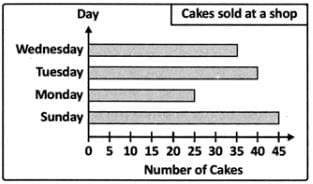Solution:
QUESTION: 8

The numbers 2, 3, 4 and 6 are all factors of which number?

Solution:

The common factors of all these numbers are 12.

QUESTION: 9

A cycle travels at a speed of 45 km/hr. How far will it travel in 36 seconds?

Solution:

45 km/h or 45000 meters ÷3600 = 12.5 meters per second x 36 seconds = 450 meters

Answer is the bus travelled a Distance of 450 meters, in Time of 36 seconds, while at Speed of 45 km/h

QUESTION: 10

The given figure shows a number line.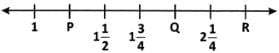Find the value of P + Q + R.

Solution:
QUESTION: 11

ABCD is a square of side 2016 cm. It is divided as shown in the figure into four equal squares. The top right hand side square is again divided into 4 equal squares. Again the top right hand square is divided into four equal squares.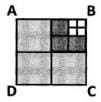Find the side of each smallest square.

Solution:
QUESTION: 12

Simplify the given expression. 3 + 7 × (5 + 1) ÷3 − 2

Solution:
QUESTION: 13

A rope of 12.6 m length is cut into 2 pieces. One piece is 3 times as long as the other. What is the length of the shorter piece (in m)?

Solution:
QUESTION: 14

What is the decimal number equivalent to 112/10 ?

Solution:
QUESTION: 15

The H.C.F. of two numbers is 28 and their LCM is 336. If one number is 112, find the other.

Solution:
QUESTION: 16

P and Q are respectively 20% and 10% lower than C, what percent of Q is P?

Solution:
QUESTION: 17

Which shape has the largest perimeter?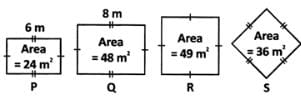Solution:
QUESTION: 18

A circle has diameter 1 m, find radius.

Solution:
QUESTION: 19

24 cups of water are needed to fill 3/5 of a basin. How many cups are needed to fill 1/2 of basin?

Solution:
QUESTION: 20

A large box contains 18 small boxes and each small box contains 25 chocolate bars. How many chocolate bars are in the large box?

Solution:

No. of Large box = 1

No. of Small box = 18

No. of Chocolate bars in each small box = 25

Total no. of Chocolate bars=25×18=450Use Code STAYHOME200 and get INR 200 additional OFF Use Coupon Code